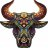# Need help with my CCI indicator#### Camelotnite

##### New member
VIP
I'm a big fan of CCI indicator. Hence I took the code and change it to a CCI indicator instead. The test results look amazing. There are some coding which I don't really understand (e.g. def CCI = 1 * (ChgRatio + 1)). Any comments will be much appreciated.

Code:
``````#######################start code
input length = 5;
input over_Bought = 100;
input over_Sold = -100;
input price = close;
input averageType = AverageType.WILDERS;
input showBreakoutSignals = no;

def NetChgAvg = MovingAverage(averageType, price - price, length);
def TotChgAvg = MovingAverage(averageType, AbsValue(price - price), length);
def ChgRatio = if TotChgAvg != 0 then NetChgAvg / TotChgAvg else 0;

def CCI = 1 * (ChgRatio + 1);
def BULL = over_Sold;
def BEAR = over_Bought;
def UpSignal = if CCI crosses above BEAR then BEAR else Double.NaN;
def DownSignal = if CCI crosses below BULL then BULL else Double.NaN;

def Bearish = CCI crosses below 100;
def Bullish = CCI crosses above -100;

#For Orders

def Ent = CCI(length)."CCI" crosses above -100 ;
def Ext = CCI(length)."CCI" crosses below 100 or CCI(length)."CCI" >= 85;

#end code``````#### horserider

##### Well-known member
VIP
That is not a CCI indicator. You are plotting the RSI and just naming it CCI.

T

#### tomsk

##### Well-known member
VIP
Here's a CCI study on the upper price chart with buy/sell arrows you might like to check out

Code:
``````input length = 8;
input trigger = 45;
input arrowdisplacer = .00075; #Change by .001 increments to move arrows
input showallarrows = no; #show only one arrow per direction or all

def price = close + low + high;
def linDev = LinDev(price, length);
def CCI = if linDev == 0
then 0
else (price - Average(price, length)) / linDev / 0.015;

def Up = CCI <= trigger and CCI > trigger;
def Down = CCI >= -trigger and CCI < -trigger;

def count = if Up == 1 and count == 0 then 1
else if count >= 1 and CCI >= -trigger then count + 1
else 0;
rec count1 = if Down == 1 and count1 == 0 then 1
else if count1 >= 1 and CCI <= trigger then count1 + 1
else 0;

plot ArrowUp = if (Up and showallarrows == no and count == 1) or (Up and showallarrows == yes)
then low * (1 - arrowdisplacer)
else Double.NaN;
ArrowUp.SetPaintingStrategy(PaintingStrategy.ARROW_UP);
ArrowUp.SetDefaultColor(Color.Yellow);
ArrowUp.SetLineWeight(3);

plot ArrowDn = if Down and count1 == 1
then high * (1 + arrowdisplacer)
else Double.NaN;
ArrowDn.SetPaintingStrategy(PaintingStrategy.ARROW_DOWN);
ArrowDn.SetDefaultColor(Color.Cyan);
ArrowDn.SetLineWeight(3);

AddLabel(yes, "CCI: " + Round(CCI, 0) + if CCI < CCI then " UP" else " DN",
if CCI > 0 then if CCI > CCI then Color.DARK_GREEN
else Color.GREEN else if CCI < CCI then Color.DARK_RED
else  Color.RED);``````

•ALV and Rojo GrandeNeed help with array variables Questions 1